# Report Sections

For most sections in a report, you can add comments in the Section properties Comments box. For some sections, you can set additional options as listed below. Many of these options are self-explanatory and are listed here only for completeness.

This table shows the report sections for each report format.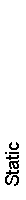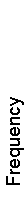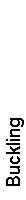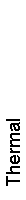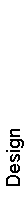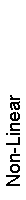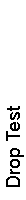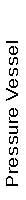Description X X X X X X X X X X Assumptions X X X X X X X X X X Model Information X X X X X X X X X X Study Properties X X X X X X X X X X Units X X X X X X X X X X Design Study Setup X Material Properties X X X X X X X X Thermal Loads X Loads & Fixtures X X X X X X Connector Definitions X X X X X Contact X X X X X X X Mesh Information X X X X X X X Sensor Results X X X X X X X Resultant Forces X X Beams X X Study Results X X X X X X X X X X Conclusion X X X X X X X X X X Appendix X X X X X X X X X X